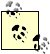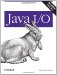# Numeric Data

Input streams read bytes and output streams write bytes. Readers read characters and writers write characters. Therefore, to understand input and output, you first need a solid understanding of how Java deals with bytes, integers, characters, and other primitive data types, and when and why one is converted into another. In many cases Java's behavior is not obvious.

1.2.1. Integer Data

The fundamental integer data type in Java is the int, a 4-byte, big-endian, two's complement integer. An int can take on all values between -2,147,483,648 and 2,147,483,647. When you type a literal integer such as 7, -8345, or 3000000000 in Java source code, the compiler treats that literal as an int. In the case of 3000000000 or similar numbers too large to fit in an int, the compiler emits an error message citing "Numeric overflow."

long s are 8-byte, big-endian, two's complement integers that range all the way from -9,223,372,036,854,775,808 to 9,223,372,036,854,775,807. long literals are indicated by suffixing the number with a lower- or uppercase L. An uppercase L is preferred because the lowercase l is too easily confused with the numeral 1 in most fonts. For example, 7L, -8345L, and 3000000000L are all 64-bit long literals.

Two more integer data types are available in Java, the short and the byte. shorts are 2-byte, big-endian, two's complement integers with ranges from -32,768 to 32,767. They're rarely used in Java and are included mainly for compatibility with C.

bytes, however, are very much used in Java. In particular, they're used in I/O. A byte is an 8-bit, two's complement integer that ranges from -128 to 127. Note that like all numeric data types in Java, a byte is signed. The maximum byte value is 127. 128, 129, and so on through 255 are not legal values for bytes.

Java has no short or byte literals. When you write the literal 42 or 24000, the compiler always reads it as an int, never as a byte or a short, even when used in the right-hand side of an assignment statement to a byte or short, like this:

```byte b = 42;
short s = 24000;
```

However, in these lines, a special assignment conversion is performed by the compiler, effectively casting the int literals to the narrower types. Because the int literals are constants known at compile time, this is permitted. However, assignments from int variables to shorts and bytes are notat least not without an explicit cast. For example, consider these lines:

```int i = 42;
byte b = i;
```

Compiling these lines produces the following errors:

```Error: Incompatible type for declaration.
Explicit cast needed to convert int to short.
ByteTest.java line 6
```

This occurs even though the compiler is theoretically capable of determining that the assignment does not lose information. To correct this, you must use explicit casts, like this:

```int i = 42;
byte b = (byte) i;
```

Even the addition of two byte variables produces an integer result and thus cannot be assigned to a byte variable without a cast. The following code produces the same error:

```byte b1 = 22;
byte b2 = 23;
byte b3 = b1 + b2;
```

For these reasons, working directly with byte variables is inconvenient at best. Many of the methods in the stream classes are documented as reading or writing bytes. However, what they really return or accept as arguments are ints in the range of an unsigned byte (0255). This does not match any Java primitive data type. These ints are then converted into bytes internally.

For instance, according to the Java class library documentation, the read( ) method of java.io.InputStream returns "the next byte of data, or -1 if the end of the stream is reached." Upon reflection, this sounds suspicious. How is a -1 that appears as part of the stream data to be distinguished from a -1 indicating end of stream? In point of fact, the read( ) method does not return a byte; its signature shows that it returns an int:

```public abstract int read( ) throws IOException
```

This int is not a Java byte with a value between -128 and 127 but a more general unsigned byte with a value between 0 and 255. Hence, -1 can easily be distinguished from valid data values read from the stream.

The write( ) method in the java.io.OutputStream class is similarly problematic. It returns void but takes an int as an argument:

```public abstract void write(int b) throws IOException
```

This int is intended to be an unsigned byte value between 0 and 255. However, there's nothing to stop a careless programmer from passing in an int value outside that range. In this case, the 8 low-order bits are written and the top 24 high-order bits are ignored:

```b = b & 0x000000FF;
```Although this is the behavior described in the Java Language Specification, since the write( ) method is abstract, actual implementation of this scheme is left to the subclasses, and a careless programmer could do something different.

On the other hand, real Java bytes are used in methods that read or write arrays of bytes. For example, consider these two read( ) methods from java.io.InputStream:

```public int read(byte[] data) throws IOException
public int read(byte[] data, int offset, int length) throws IOException
```

While the difference between an 8-bit byte and a 32-bit int is insignificant for a single number, it can be very significant when several thousand to several million numbers are read. In fact, a single byte still takes up four bytes of space inside the Java virtual machine, but a byte array occupies only the amount of space it actually needs. The virtual machine includes special instructions for operating on byte arrays but does not include any instructions for operating on single bytes. They're just promoted to ints.

Although data is stored in the array as signed Java bytes with values between -128 and 127, there's a simple one-to-one correspondence between these signed values and the unsigned bytes normally used in I/O. This correspondence is given by the following formula:

```int unsignedByte = signedByte >= 0 ? signedByte : 256 + signedByte;
```

1.2.2. Conversions and Casts

Since bytes have such a small range, they're often converted to ints in calculations and method invocations. Often, they need to be converted back, generally through a cast. Therefore, it's useful to have a good grasp of exactly how the conversion occurs.

Casting from an int to a bytefor that matter, casting from any wider integer type to a narrower typetakes place through truncation of the high-order bytes. This means that as long as the value of the wider type can be expressed in the narrower type, the value is not changed. The int 127 cast to a byte still retains the value 127.

On the other hand, if the int value is too large for a byte, strange things happen. The int 128 cast to a byte is not 127, the nearest byte value. Instead, it is -128. This occurs through the wonders of two's complement arithmetic. Written in hexadecimal, 128 is 0x00000080. When that int is cast to a byte, the leading zeros are truncated, leaving 0x80. In binary, this can be written as 10000000. If this were an unsigned number, 10000000 would be 128 and all would be fine, but this isn't an unsigned number. Instead, the leading bit is a sign bit, and that 1 does not indicate 27 but a minus sign. The absolute value of a negative number is found by taking the complement (changing all the 1 bits to 0 bits and vice versa) and adding 1. The complement of 10000000 is 01111111. Adding 1, you have 01111111 + 1 = 10000000 = 128 (decimal). Therefore, the byte 0x80 actually represents -128. Similar calculations show that the int 129 is cast to the byte -127, the int 130 is cast to the byte -126, the int 131 is cast to the byte -125, and so on. This continues through the int 255, which is cast to the byte -1.In this book, as in Java source code, all numbers preceded by 0x are read as hexadecimal.

When 256 is reached, the low-order bytes of the int are filled with zeros. In other words, 256 is 0x00000100. Thus, casting it to a byte produces 0, and the cycle starts over. This behavior can be reproduced algorithmically with this formula, though a cast is obviously simpler:

```int byteValue;
int temp = intValue % 256;
if ( intValue < 0) {
byteValue = temp < -128 ? 256 + temp : temp;
}
else {
byteValue = temp > 127 ? temp - 256 : temp;
}
```Java I/O
ISBN: 0596527500
EAN: 2147483647
Year: 2004
Pages: 244